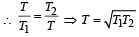Courses

# 28 Year NEET Questions: Thermodynamics- 1

## 14 Questions MCQ Test Physics Class 11 | 28 Year NEET Questions: Thermodynamics- 1

Description
This mock test of 28 Year NEET Questions: Thermodynamics- 1 for NEET helps you for every NEET entrance exam. This contains 14 Multiple Choice Questions for NEET 28 Year NEET Questions: Thermodynamics- 1 (mcq) to study with solutions a complete question bank. The solved questions answers in this 28 Year NEET Questions: Thermodynamics- 1 quiz give you a good mix of easy questions and tough questions. NEET students definitely take this 28 Year NEET Questions: Thermodynamics- 1 exercise for a better result in the exam. You can find other 28 Year NEET Questions: Thermodynamics- 1 extra questions, long questions & short questions for NEET on EduRev as well by searching above.
QUESTION: 1

### In thermodynamic processes which of the following statements is not true? 

Solution:

In an isochoric process volume remains constant whereas pressure remains constant in isobaric process.

QUESTION: 2

### The internal energy change in a system that has absorbed 2 kcals of heat and done 500 J of work is: 

Solution:

According to first law of thermodynamics
Q = ΔU + W
ΔU = Q – W
= 2 × 4.2 × 1000 – 500 = 8400 –500
= 7900 J

QUESTION: 3

### If ΔU and ΔW represent the increase in internal energy and work done by the system respectively in a thermodynamical process, which of the following is true? 

Solution:

By first law of thermodynamics,
ΔQ = ΔU + ΔW
In adiabatic process, ΔQ = 0
∴  ΔU = -ΔW
In isothermal process, ΔU = 0
∴  ΔQ -ΔW

QUESTION: 4

During an isothermal expansion, a confined ideal gas does –150 J of work against its surroundings. This implies that 

Solution:

From the first law of thermodynamics
ΔU = Q + W
For isothermal process, ΔU = 0
Therefore, Q = - W
Given W = - 150
Therefore, Q = + 150
When Q is positive, the heat is added to the gas.

QUESTION: 5

When 1 kg of ice at 0°C melts to water at 0°C, the resulting change in its entropy, taking latent heat of ice to be 80 cal/°C, is 

Solution:

Change in entropy is given by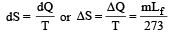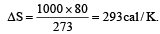QUESTION: 6

A mass of diatomic gas (γ= 1.4) at a pressure of 2 atmospheres is compressed adiabatically so that its temperature rises from 27°C to 927°C.The pressure of the gas in final state is [2011M]

Solution:

T1 = 273 + 27 = 300K
T2 = 273 + 927 = 1200K
For adiabatic process,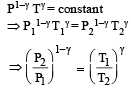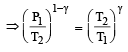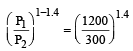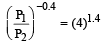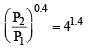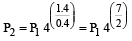= P1 (27) = 2 × 128 = 256 atm

QUESTION: 7

A thermodynamic system is taken through the cycle ABCD as shown in figure. Heat rejected by the gas during the cycle is :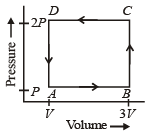Solution:

∵ Internal energy is the state function.
∴ In cyclie process; ΔU = 0
According to 1st law of thermodynamics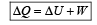So heat absorbed ΔQ = W = Area under the curve
= –  (2V) (P) = –  2PV
So heat rejected  =  2PV

QUESTION: 8

One mole of an ideal gas goes from an initial state A to final state B via two processes : It first undergoes isothermal expansion from volume V to 3V and then its volume is reduced from 3V to V at constant pressure. The correct P-V diagram representing the two processes is : 

Solution:

1st process is isothermal expansion which is only correct shown in option (d) 2nd process is isobaric compression which is correctly shown in option (d)

QUESTION: 9

If Q1, Q2, Q3 indicate the heat a absorbed by the gas along the three processes and ΔU1, ΔU2, ΔU3 indicate the change in internal energy along the three processes respectively, then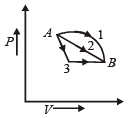Solution:

Initial and final condition is same for all process ΔU1 =  ΔU2 = ΔU3
from first law of thermodynamics ΔQ = ΔU + ΔW
Work done ΔW1 > ΔW2 > ΔW3 (Area of P.V. graph)
So ΔQ1 > ΔQ2 > ΔQ3

QUESTION: 10

A gas is taken through the cycle A → B → C → A, as shown in figure. What is the net work done by the gas ? [NEET 2013]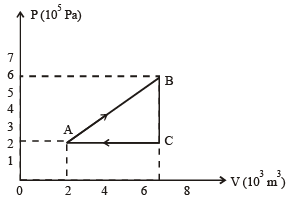Solution:

Wnet = Area of triangle ABC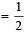AC × BC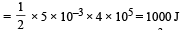QUESTION: 11

During an adiabatic process, the pressure of a gas is found to be proportional to the cube of its temperature. The ratio of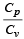for the gas is   [NEET 2013]

Solution:

According to question P ∝ T3
But as we know for an adiabatic process the pressure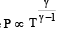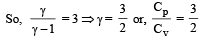QUESTION: 12

A system is taken from state a to state c by two paths adc and abc as shown in the figure. The internal energy at a is Ua = 10 J. Along the path adc the amount of heat absorbed δQ1 = 50 J and the work done δW1 = 20 J whereas along the path abc the heat absorbed δQ2 = 36 J. The amount of work done along the path abc is [NEET Kar. 2013]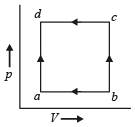Solution:

From first law of thermodynamics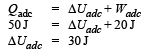Again,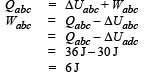QUESTION: 13

Which of the following relations does not give the equation of an adiabatic process, where terms have their usual meaning? [NEET Kar. 2013]

Solution:

Adiabatic equations of state are:
PVγ = constant
TVγ–1 = constant
P1–γTγ = constant.

QUESTION: 14

Two Carnot engines A and B are operated in series. The engine A receives heat from the source at temperature T1 and rejects the heat to the sink at temperature T. The second engine B receives the heat at temperature T and rejects to its sink at temperature T2. For what value of T the efficiencies of the two engines are equal? [NEET Kar. 2013]

Solution:

Efficiency of engine A,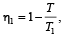Efficiency of engine B,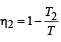Here, η1 = η2